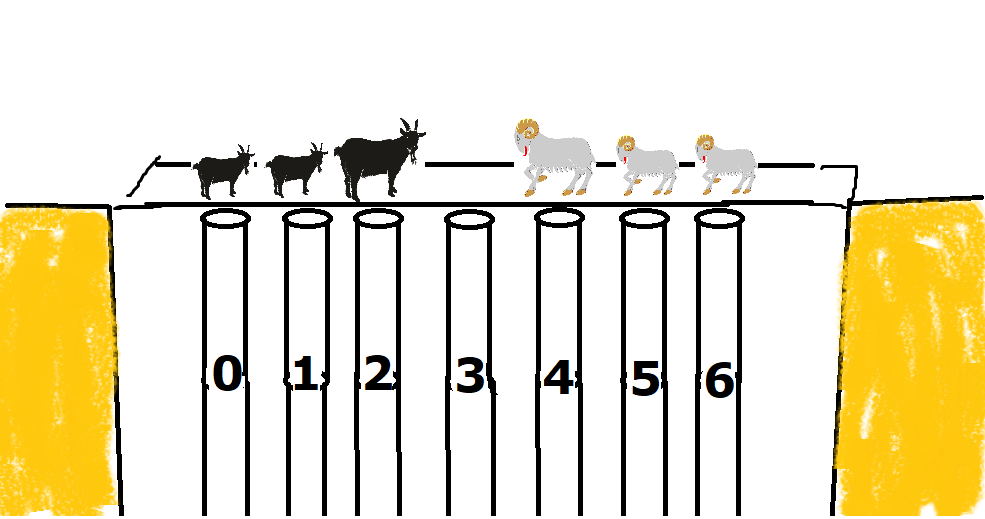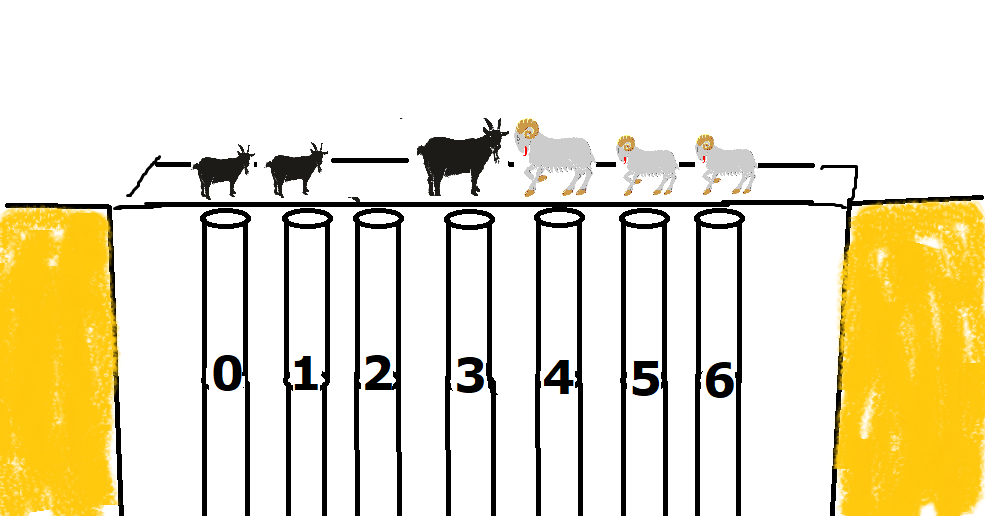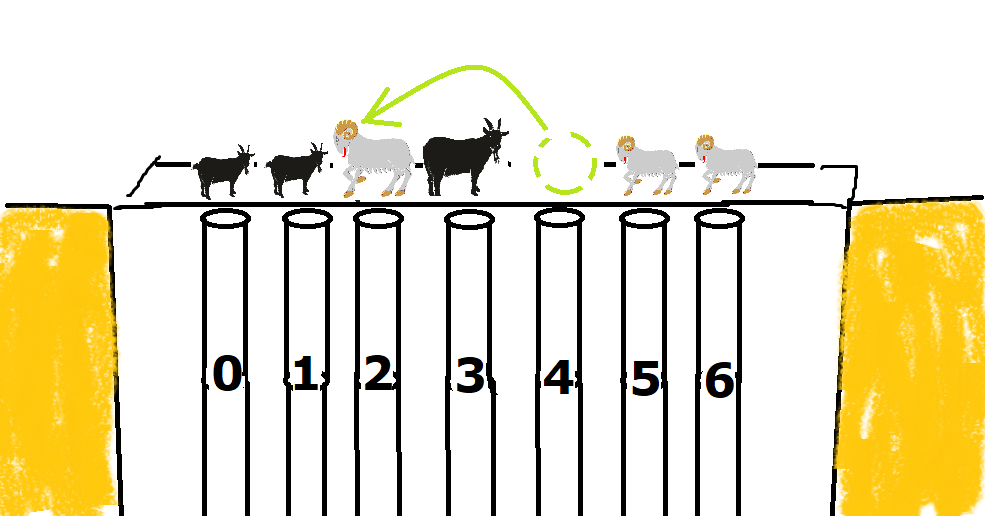#DAY 28
3
Software Development

## 【小白馬的程式大冒險】Day28- 黑白羊爭過河，無可奈何之橋

(注意: 本劇本有許多天馬行空的元素，喜歡看傳統教科書者請斟酌閱讀)

## 小白馬的第二試煉-續

``````input: The□□□secret□□□□password□□□is□□□□□5678.
output: 3435 (中間空白數量依序為3、4、3、5)
``````

``````Str = "The   secret    password   is     5678."
return Str.split()

``````## 圖地反轉的靈閃

``````Str = "The   secret    password   is     5678."
trans= ''.join(['A' if c==' ' else ' ' for c in Str])
return list(map(len, trans.split()))

``````

``````也就是說，原來字串是

AAA      AAAA        AAA  AAAAA
``````

# 第二章- 無可奈何橋## 彷彿若有光

「復前行，欲窮其林。林盡水源，便得一山。山有小口，彷彿若有光。」

``````當你打開這紙條時，想必你已經來到無可奈何橋了，

1. 向前一格走到前方空格
2. 跳過對方一隻羊進入前面的一個空位(不可跳過兩隻羊)

``````「馬兄，十五分鐘給出解OK?」

## n皇后問題的遞迴靈感

``````def goatmove(goats, move=()):
if goats 達到終止條件:
return [move]
ans = []
for 所有山羊可能的移動:
ans += goatmove(移動後的goats, move+(某個山羊移動))
``````

``````def goatmove(goats, move=()):
if goats==[-1,-1,-1,0,1,1,1]: #遞迴終止條件
return [move]
ans = []
length = len(goats)
for i in range(length): #檢查每隻羊是否能動
if i<length-2 and goats[i]==1 and goats[i+1]==-1 and goats[i+2]==0: #向右跳
new_goats = goats[:]
new_goats[i], new_goats[i+2] = new_goats[i+2], new_goats[i]
ans+= goatmove(new_goats, move+(i,"向右跳"))
if i>1 and goats[i-2]==0 and goats[i-1]==1 and goats[i]==-1: #向左跳
new_goats = goats[:]
new_goats[i], new_goats[i-2] = new_goats[i-2], new_goats[i]
ans+= goatmove(new_goats, move+(i,"向左跳"))
if i<length-1 and goats[i]==1 and goats[i+1]==0: #向右走
new_goats = goats[:]
new_goats[i], new_goats[i+1] = new_goats[i+1], new_goats[i]
ans+= goatmove(new_goats, move+(i,"向右走"))
if i>0 and goats[i-1]==0 and goats[i]==-1: #向左走
new_goats = goats[:]
new_goats[i], new_goats[i-1] = new_goats[i-1], new_goats[i]
ans+= goatmove(new_goats, move+(i,"向左走"))
return ans

goats = [1,1,0,1,-1,-1,-1]
print(goatmove(goats))
``````

## 指引過橋「白羊 白羊 白羊 空格 黑羊 黑羊 黑羊」了。